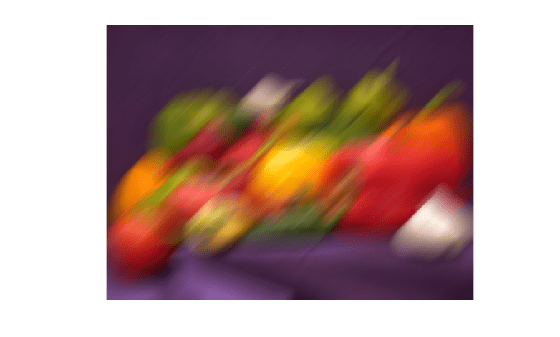Documentation

### This is machine translation

Mouseover text to see original. Click the button below to return to the English version of the page.

# imfilter

N-D filtering of multidimensional images

## Syntax

``B = imfilter(A,h)``
``B = imfilter(A,h,options,...)``

## Description

example

````B = imfilter(A,h)` filters the multidimensional array `A` with the multidimensional filter `h` and returns the result in `B`.You optionally can filter a multidimensional array with a 2-D filter using a GPU (requires Parallel Computing Toolbox™). For more information, see Image Processing on a GPU.```

example

````B = imfilter(A,h,options,...)` performs multidimensional filtering according to one or more specified options.```

## Examples

collapse all

Read a color image into the workspace and display it.

```originalRGB = imread('peppers.png'); imshow(originalRGB)```Create a motion-blur filter using the `fspecial` function.

`h = fspecial('motion', 50, 45);`

Apply the filter to the original image to create an image with motion blur. Note that `imfilter` is more memory efficient than some other filtering functions in that it outputs an array of the same data type as the input image array. In this example, the output is an array of `uint8`.

```filteredRGB = imfilter(originalRGB, h); figure, imshow(filteredRGB)```Filter the image again, this time specifying the replicate boundary option.

```boundaryReplicateRGB = imfilter(originalRGB, h, 'replicate'); figure, imshow(boundaryReplicateRGB)```By default, `imfilter` uses correlation because the toolbox filter design functions produce correlation kernels. Use the optional parameter to use convolution.

Create a sample matrix.

`A = magic(5)`
```A = 5×5 17 24 1 8 15 23 5 7 14 16 4 6 13 20 22 10 12 19 21 3 11 18 25 2 9 ```

Create a filter.

`h = [-1 0 1];`

Filter using correlation, the default.

`imfilter(A,h)`
```ans = 5×5 24 -16 -16 14 -8 5 -16 9 9 -14 6 9 14 9 -20 12 9 9 -16 -21 18 14 -16 -16 -2 ```

Filter using convolution, specifying `imfilter` with the optional parameter.

`imfilter(A,h,'conv')`
```ans = 5×5 -24 16 16 -14 8 -5 16 -9 -9 14 -6 -9 -14 -9 20 -12 -9 -9 16 21 -18 -14 16 16 2 ```

In this example, the output of `imfilter` has negative values when the input is of class `double`. To avoid negative values, convert the image to a different data type before calling `imfilter`. For example, when the input type is `uint8`, `imfilter` truncates output values to `0`. It might also be appropriate to convert the image to a signed integer type.

`A = magic(5)`
```A = 5×5 17 24 1 8 15 23 5 7 14 16 4 6 13 20 22 10 12 19 21 3 11 18 25 2 9 ```

Filter the image with `imfilter`.

```h = [-1 0 1]; imfilter(A,h)```
```ans = 5×5 24 -16 -16 14 -8 5 -16 9 9 -14 6 9 14 9 -20 12 9 9 -16 -21 18 14 -16 -16 -2 ```

Notice that the result has negative values. To avoid negative values in the output image, convert the input image to `uint8` before performing the filtering. Since the input to `imfilter` is of class `uint8`, the output also is of class `uint8`, and `imfilter` truncates negative values to `0`.

```A = uint8(magic(5)); imfilter(A,h)```
```ans = 5x5 uint8 matrix 24 0 0 14 0 5 0 9 9 0 6 9 14 9 0 12 9 9 0 0 18 14 0 0 0 ```

## Input Arguments

collapse all

Image to be filtered, specified as a numeric array of dimension.

Data Types: `single` | `double` | `int8` | `int16` | `int32` | `uint8` | `uint16` | `uint32` | `logical`

Multidimensional filter, specified as an N-D array of data type `double`.

Data Types: `double`

Options that control the filtering operation, specified as a character vector, string scalar, or numeric scalar. The following table lists all supported options.

Boundary Options

Option

Description

numeric scalar, `X`

Input array values outside the bounds of the array are assigned the value `X`. When no padding option is specified, the default is `0`.

`'symmetric'`

Input array values outside the bounds of the array are computed by mirror-reflecting the array across the array border.

`'replicate'`

Input array values outside the bounds of the array are assumed to equal the nearest array border value.

`'circular'`

Input array values outside the bounds of the array are computed by implicitly assuming the input array is periodic.

Output Size

`'same'`

The output array is the same size as the input array. This is the default behavior when no output size options are specified.

`'full'`

The output array is the full filtered result, and so is larger than the input array.

Correlation and Convolution Options

`'corr'`

`imfilter` performs multidimensional filtering using correlation, which is the same way that `filter2` performs filtering. When no correlation or convolution option is specified, `imfilter` uses correlation.

`'conv'`

`imfilter` performs multidimensional filtering using convolution.

## Output Arguments

collapse all

Filtered image, returned as a numeric array of the same size and class as the input image, `A`.

## Tips

• This function may take advantage of hardware optimization for data types `uint8`, `uint16`, `int16`, `single`, and `double` to run faster.

## Algorithms

• The `imfilter` function computes the value of each output pixel using double-precision, floating-point arithmetic. If the result exceeds the range of the data type, then `imfilter` truncates the result to the allowed range of the data type. If it is an integer data type, then `imfilter` rounds fractional values.

• If you specify an even-sized kernel `h`, then the center of the kernel is `floor((size(h) + 1)/2)`.

For example, the center of 4-element filter `[0.25 0.75 -0.75 -0.25]` is the second element, `0.75`. This filter gives identical results as filtering with the 5-element filter `[0 0.25 0.75 -0.75 -0.25]`.